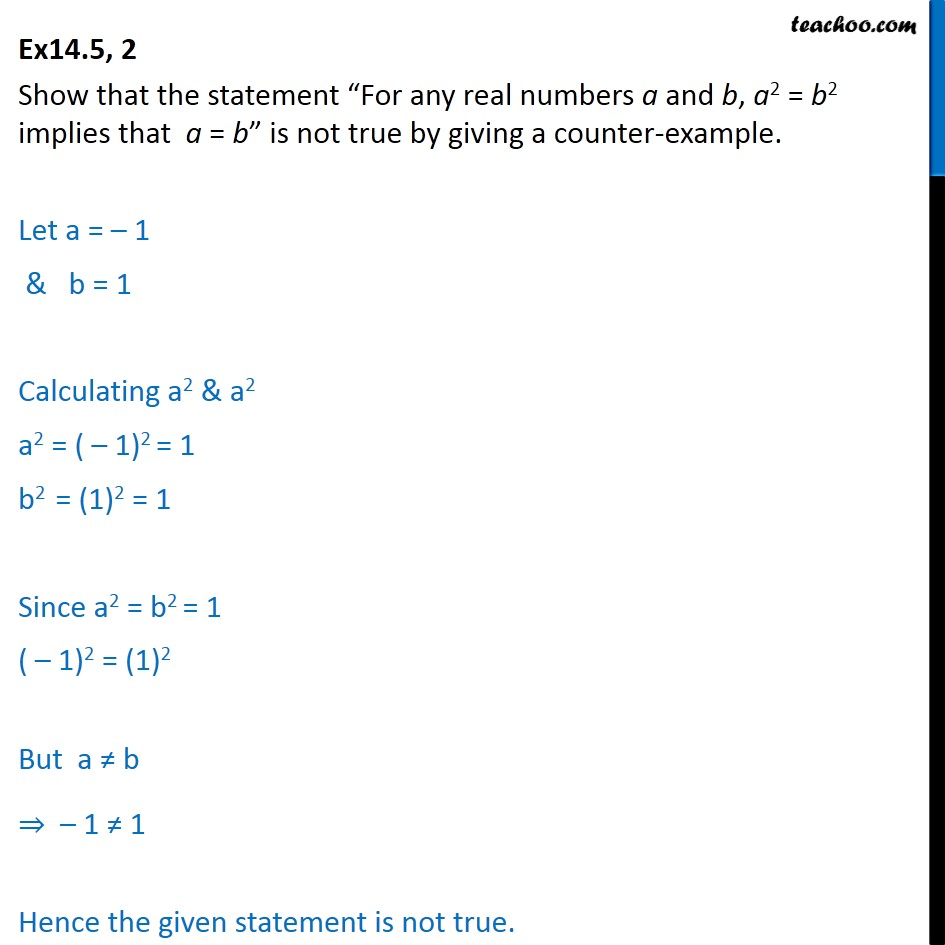Proving Statements true or false

Mathematical Reasoning
Serial order wiseLearn in your speed, with individual attention - Teachoo Maths 1-on-1 Class

### Transcript

Question 2 Show that the statement “For any real numbers a and b, a2 = b2 implies that a = b” is not true by giving a counter-example. Let a = – 1 & b = 1 Calculating a2 & a2 a2 = ( – 1)2 = 1 b2 = (1)2 = 1 Since a2 = b2 = 1 ( – 1)2 = (1)2 But a ≠ b ⇒ – 1 ≠ 1 Hence the given statement is not true.